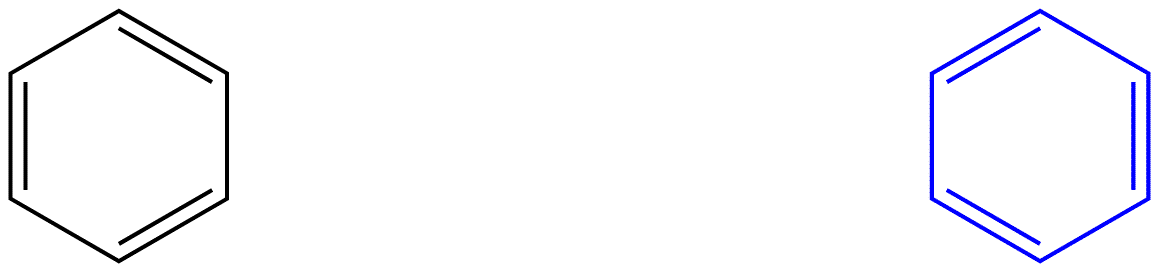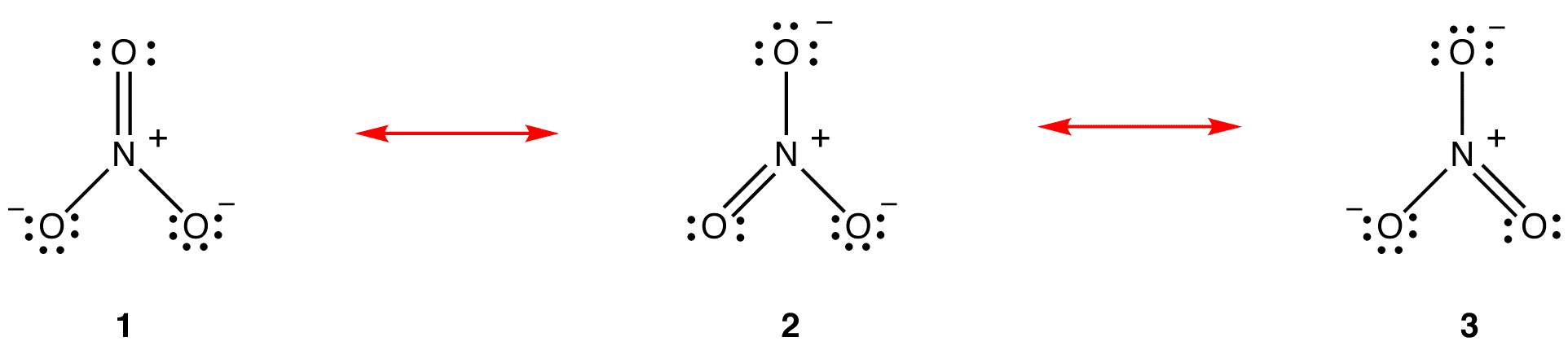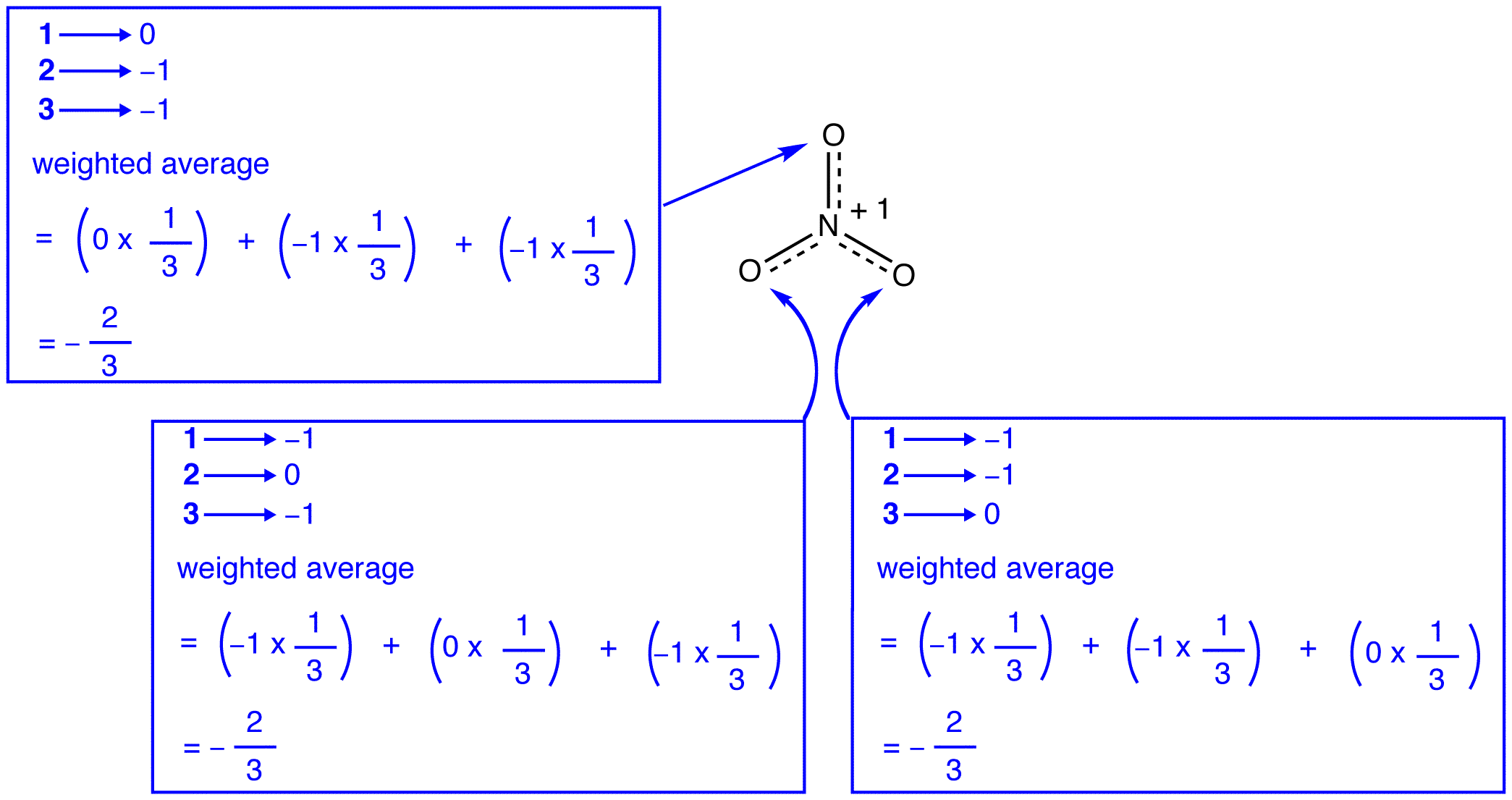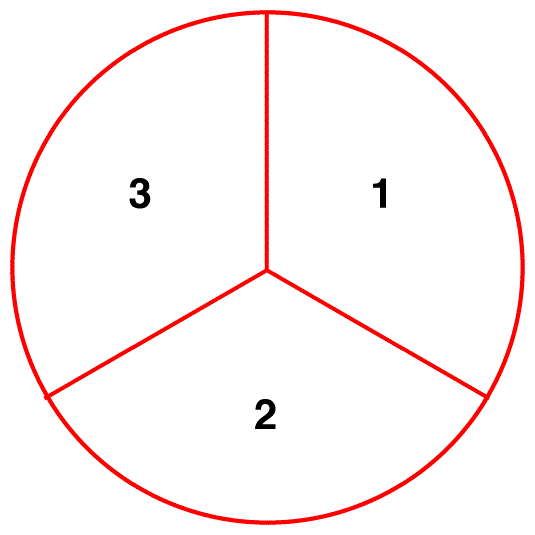# 12.7: Resonance and Electron Delocalization

$$\newcommand{\vecs}{\overset { \rightharpoonup} {\mathbf{#1}} }$$ $$\newcommand{\vecd}{\overset{-\!-\!\rightharpoonup}{\vphantom{a}\smash {#1}}}$$$$\newcommand{\id}{\mathrm{id}}$$ $$\newcommand{\Span}{\mathrm{span}}$$ $$\newcommand{\kernel}{\mathrm{null}\,}$$ $$\newcommand{\range}{\mathrm{range}\,}$$ $$\newcommand{\RealPart}{\mathrm{Re}}$$ $$\newcommand{\ImaginaryPart}{\mathrm{Im}}$$ $$\newcommand{\Argument}{\mathrm{Arg}}$$ $$\newcommand{\norm}{\| #1 \|}$$ $$\newcommand{\inner}{\langle #1, #2 \rangle}$$ $$\newcommand{\Span}{\mathrm{span}}$$ $$\newcommand{\id}{\mathrm{id}}$$ $$\newcommand{\Span}{\mathrm{span}}$$ $$\newcommand{\kernel}{\mathrm{null}\,}$$ $$\newcommand{\range}{\mathrm{range}\,}$$ $$\newcommand{\RealPart}{\mathrm{Re}}$$ $$\newcommand{\ImaginaryPart}{\mathrm{Im}}$$ $$\newcommand{\Argument}{\mathrm{Arg}}$$ $$\newcommand{\norm}{\| #1 \|}$$ $$\newcommand{\inner}{\langle #1, #2 \rangle}$$ $$\newcommand{\Span}{\mathrm{span}}$$$$\newcommand{\AA}{\unicode[.8,0]{x212B}}$$

Resonance is a mental exercise and method within the Valence Bond Theory of bonding that describes the delocalization of electrons within molecules. It compares and contrasts two or more possible Lewis structures that can represent a particular molecule. Resonance structures are used when one Lewis structure for a single molecule cannot fully describe the bonding that takes place between neighboring atoms relative to the empirical data for the actual bond lengths between those atoms. The net sum of valid resonance structures is defined as a resonance hybrid, which represents the overall delocalization of electrons within the molecule. A molecule that has several resonance structures is more stable than one with fewer. Some resonance structures are more favorable than others.

## Introduction

Electrons have no fixed position in atoms, compounds and molecules (see image below) but have probabilities of being found in certain spaces (orbitals). Resonance forms illustrate areas of higher probabilities (electron densities). This is like holding your hat in either your right hand or your left. The term Resonance is applied when there are two or more possibilities available. Chemists use Lewis diagrams to depict structure and bonding of covalent entities, such as molecules and polyatomic ions, henceforth, molecules. The Lewis diagram of many a molecule, however, is not consistent with the observed properties of the molecule.

Example $$\PageIndex{1}$$: Nitrate Ion

The nitrate ion, according to its Lewis diagram, has two types of nitrogen-oxygen bonds, one double bond and two single bonds, suggesting that one nitrogen-oxygen bond in the nitrate ion is shorter and stronger than each of the other two. Also, the Lewis structure implies, with respect to formal charge, that there are two types of oxygen atoms in the nitrate ion, one formally neutral and each of the other two bearing a formal charge of –1. Experimentally, however, the three nitrogen-oxygen bonds in the nitrate ion have the same bond length and the same bond energy, and the three oxygen atoms are indistinguishable. The Lewis diagram fails to explain the structure and bonding of the nitrate ion satisfactorily.

Two additional Lewis diagrams can be drawn for the nitrate ion.However, none of them are consistent with the observed properties of the nitrate ion and, therefore, does not correctly depict the nitrate ion.

Example $$\PageIndex{2}$$: Benzene

Benzene, according to its Lewis diagram, has two types of carbon-carbon bonds, three double bonds and three single bonds, suggesting that each of three carbon-carbon bonds in benzene is shorter and stronger than each of the other three. Experimentally, however, the six carbon-carbon bonds in benzene have the same bond length and the same bond energy. The Lewis diagram fails to explain the structure and bonding of benzene satisfactorily.

An attribute of molecules of which the classical Lewis diagram is not consistent with the observed properties is that other valid Lewis diagrams can be generated for them. One additional Lewis diagram can be drawn for benzene.However, none of them are consistent with the observed properties of benzene and, therefore, does not correctly depict benzene.

Resonance theory is an attempt to explain the structure of a species, like the nitrate ion or benzene, no Lewis diagram of which is consistent with the observed properties of the species. The major advantage of resonance theory is that, although based on rigorous mathematical analysis, resonance theory can be applied successfully invoking little or no math. Resonance theory is explained below using the nitrate ion as the example.

According to resonance theory, the structure of the nitrate ion is not 1 nor 2 nor 3 but the average of all three of them, weighted by stability. Lewis diagrams 1, 2, and 3 are called resonance forms, resonance structures, or resonance contributors of the nitrate ion. The structure of the nitrate ion is said to be a resonance hybrid or, simply, hybrid of resonance forms 1, 2, and 3. Whenever it is necessary to show the structure of the nitrate ion, resonance forms 1, 2, and 3 are drawn, connected by a double-headed arrows.The three resonance forms of the nitrate ion, 1, 2, and 3, are identical, so they have the same stability and, therefore, contribute equally to the hybrid. Since the exact extent to which each resonance form of the nitrate ion contributes to the hybrid is known, the bond order of each nitrogen-oxygen bond as well as the formal charge on each oxygen atom in the hybrid can be easily determined:According to resonance theory, each bond in the nitrate ion is one and one-third of a bond, which is consistent with the observation that the three bonds in the nitrate ion have the same bond length and the same bond energy.According to resonance theory, each oxygen atom in the nitrate ion has a formal charge of –2/3, which, in conjunction with the fact that the three nitrogen-oxygen bonds are identical, is consistent with the observation that the three oxygen atoms in the nitrate ion are indistinguishable. In each resonance form of the nitrate ion, there are two $$\pi$$ electrons, and they are shared only by two atoms. An electron shared only by two atoms is said to be localized. Thus, the two $$\pi$$ electrons in each resonance form of the nitrate ion are localized. The nitrate ion, as represented by the hybrid, has two $$\pi$$ electrons:

• # electrons in one $$\pi$$ bond = 2
• # electrons in one-third of a $$\pi$$ bond = 2/3
• # electrons in three of them = 3 x (2/3) = 2

The two $$\pi$$ electrons in the nitrate ion are shared by a total of four atoms, one nitrogen atom and three oxygen atoms. An electron shared by more than two atoms is said to be delocalized. Thus, the two $$\pi$$ electrons in the nitrate ion are delocalized. Delocalization of $$\pi$$ electrons in the nitrate ion requires that the four atoms be on the same plane, allowing lateral overlap of the p orbitals on them.If the energy of the nitrate ion were the weighted average of the energies of its three resonance forms, just as the structure of the nitrate ion is the weighted average of the structures of its three resonance forms, it should be equal to the energy of one of the three identical resonance forms:If the energy of the hybrid were equal to that of a resonance form, given that all chemical entities (elementary particles, atoms, molecules, etc.) naturally tend to be in the lowest possible energy state, there would be no advantage for the nitrate ion to exist as the hybrid; it could simply exist as a resonance form. Since the nitrate ion exists as the hybrid, not as a resonance form, it can be inferred that the energy of the hybrid is lower than that of any of the resonance forms.According to resonance theory then, the energy of a molecule is lower than that of the lowest-energy resonance form. Since the nitrate ion has lower energy and, therefore, is more stable than any of its resonance forms, the nitrate ion is said to be resonance stabilized.

Common Misconceptions

There are two misconceptions about resonance theory among beginning students, likely due to literal interpretation of the word resonance. They are described below, using the nitrate ion as the example.

Misconception 1: The nitrate ion exists as resonance form 1 for a moment and then changes either to resonance form 2 or to resonance form 3, which interconvert, or revert to 1.The structure of the nitrate ion is not 1 nor 2 nor 3 but the hybrid and does not change with time unless undergoing a reaction.

Misconception 2: In a sample of nitrate ions, at a given moment, one-third of the ions exist as resonance form 1, another one-third as resonance form 2, and the remaining one-third as resonance form 3.In a sample of nitrate ions, at a given moment, all ions have the same structure, which is the hybrid.

Mules and Rhinoceros

The classic analogy used to clarify these two misconceptions is the mule (Morrison, R. T.; Boyd, R.N. Organic Chemistry, fifth edition; Allyn and Bacon: Boston, 1987, pg. 373). Biologically, a mule is a hybrid of a horse and a donkey. This does not mean that a mule resembles a horse for a moment and then changes to resemble a donkey. The appearance of a mule is a combination of that of a horse and that of a donkey and does not change with time. Nor does it mean that, in a herd, some mules resemble a horse and the others a donkey. In a herd, all mules have the same appearance, which is a combination of a horse and a donkey. The weakness of this analogy is that horses and donkeys do exist, whereas resonance forms are strictly hypothetical. A better analogy, cited in Morrison and Boyd, is the rhinoceros. Upon seeing a rhinoceros, one could describe it as the hybrid of a dragon and a unicorn, two creatures that do not exist.

## Peptide Bonds

A consideration of resonance contributors is crucial to any discussion of the amide functional group. One of the most important examples of amide groups in nature is the ‘peptide bond’ that links amino acids to form polypeptides and proteins.Critical to the structure of proteins is the fact that, although it is conventionally drawn as a single bond, the C-N bond in a peptide linkage has a significant barrier to rotation, almost as if it were a double bond.This, along with the observation that the bonding around the peptide nitrogen has trigonal planar geometry, strongly suggests that the nitrogen is sp2-hybridized. An important resonance contributor has a C=N double bond and a C-O single bond, with a separation of charge between the oxygen and the nitrogen.Although B is a minor contributor due to the separation of charges, it is still very relevant in terms of peptide and protein structure – our proteins would simply not fold up properly if there was free rotation about the peptide C-N bond.